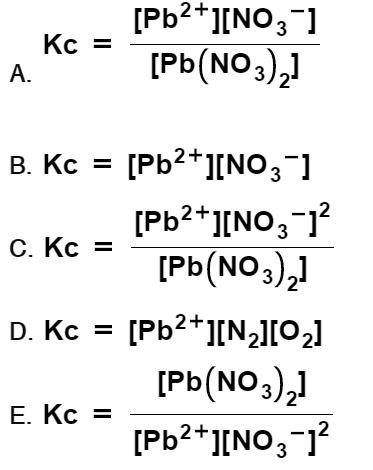# Problem: Which is the correct Kc expression for the following equilibrium reaction?Pb(NO3)2(aq) ⇌ Pb2+(aq) + 2 NO3–(aq)

###### FREE Expert Solution

Setup the Kc expression for the reaction and determine which is the correct one for the reaction:

Pb(NO3)2(aq)  Pb2+(aq) + 2 NO3(aq)

Kc is the equilibrium expression of the concentrations in the reaction. The general form of an equilibrium expression is:

87% (220 ratings)###### Problem Details

Which is the correct Kc expression for the following equilibrium reaction?

Pb(NO3)2(aq) ⇌ Pb2+(aq) + 2 NO3(aq)